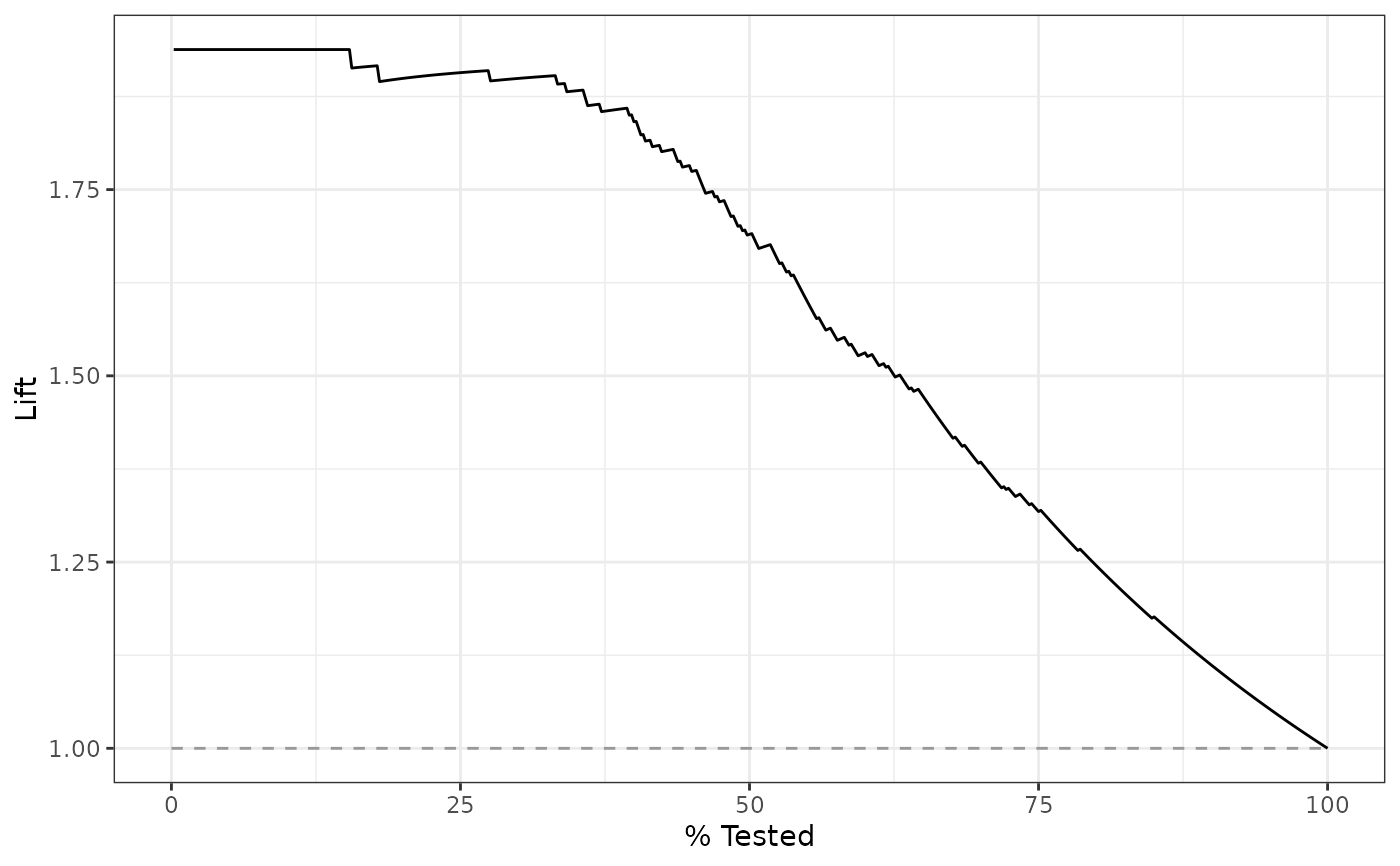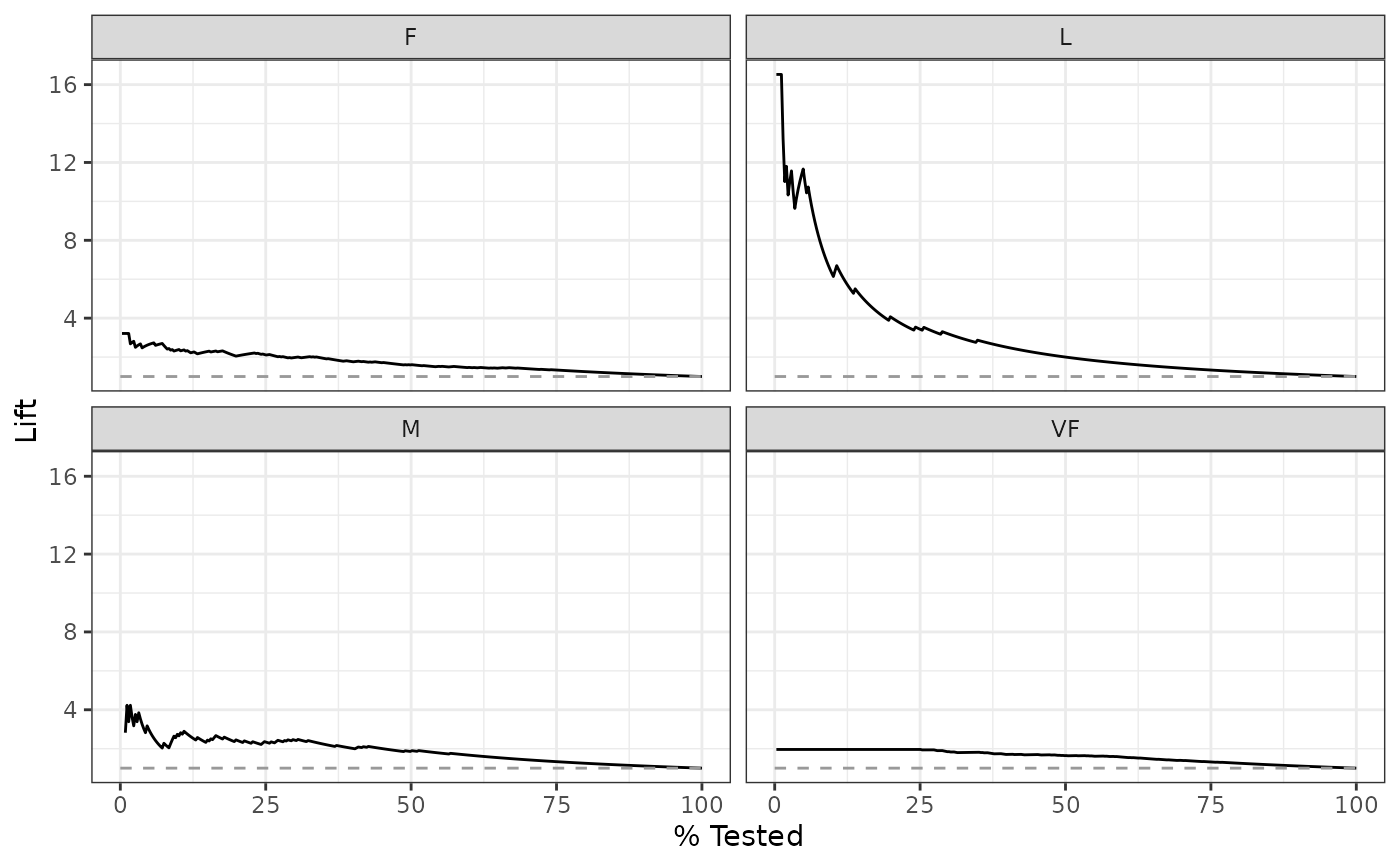lift_curve() constructs the full lift curve and returns a tibble. See gain_curve() for a closely related concept.

## Usage

lift_curve(data, ...)

# S3 method for data.frame
lift_curve(
data,
truth,
...,
na_rm = TRUE,
event_level = yardstick_event_level(),
case_weights = NULL
)

## Arguments

data

A data.frame containing the columns specified by truth and ....

...

A set of unquoted column names or one or more dplyr selector functions to choose which variables contain the class probabilities. If truth is binary, only 1 column should be selected. Otherwise, there should be as many columns as factor levels of truth.

truth

The column identifier for the true class results (that is a factor). This should be an unquoted column name although this argument is passed by expression and supports quasiquotation (you can unquote column names). For _vec() functions, a factor vector.

na_rm

A logical value indicating whether NA values should be stripped before the computation proceeds.

event_level

A single string. Either "first" or "second" to specify which level of truth to consider as the "event". This argument is only applicable when estimator = "binary". The default uses an internal helper that generally defaults to "first", however, if the deprecated global option yardstick.event_first is set, that will be used instead with a warning.

case_weights

The optional column identifier for case weights. This should be an unquoted column name that evaluates to a numeric column in data. For _vec() functions, a numeric vector.

## Value

A tibble with class lift_df or lift_grouped_df having columns:

• .n The index of the current sample.

• .n_events The index of the current unique sample. Values with repeated estimate values are given identical indices in this column.

• .percent_tested The cumulative percentage of values tested.

• .lift First calculate the cumulative percentage of true results relative to the total number of true results. Then divide that by .percent_tested.

If using the case_weights argument, all of the above columns will be weighted. This makes the most sense with frequency weights, which are integer weights representing the number of times a particular observation should be repeated.

## Details

There is a ggplot2::autoplot() method for quickly visualizing the curve. This works for binary and multiclass output, and also works with grouped data (i.e. from resamples). See the examples.

## Gain and Lift Curves

The motivation behind cumulative gain and lift charts is as a visual method to determine the effectiveness of a model when compared to the results one might expect without a model. As an example, without a model, if you were to advertise to a random 10% of your customer base, then you might expect to capture 10% of the of the total number of positive responses had you advertised to your entire customer base. Given a model that predicts which customers are more likely to respond, the hope is that you can more accurately target 10% of your customer base and capture >10% of the total number of positive responses.

The calculation to construct lift curves is as follows:

1. truth and estimate are placed in descending order by the estimate values (estimate here is a single column supplied in ...).

2. The cumulative number of samples with true results relative to the entire number of true results are found.

3. The cumulative % found is divided by the cumulative % tested to construct the lift value. This ratio represents the factor of improvement over an uninformed model. Values >1 represent a valuable model. This is the y-axis of the lift chart.

## Multiclass

If a multiclass truth column is provided, a one-vs-all approach will be taken to calculate multiple curves, one per level. In this case, there will be an additional column, .level, identifying the "one" column in the one-vs-all calculation.

## Relevant Level

There is no common convention on which factor level should automatically be considered the "event" or "positive" result when computing binary classification metrics. In yardstick, the default is to use the first level. To alter this, change the argument event_level to "second" to consider the last level of the factor the level of interest. For multiclass extensions involving one-vs-all comparisons (such as macro averaging), this option is ignored and the "one" level is always the relevant result.

Other curve metrics: gain_curve(), pr_curve(), roc_curve()

Max Kuhn

## Examples

# ---------------------------------------------------------------------------
# Two class example

# truth is a 2 level factor. The first level is "Class1", which is the
# "event of interest" by default in yardstick. See the Relevant Level
# section above.
data(two_class_example)

# Binary metrics using class probabilities take a factor truth column,
# and a single class probability column containing the probabilities of
# the event of interest. Here, since "Class1" is the first level of
# "truth", it is the event of interest and we pass in probabilities for it.
lift_curve(two_class_example, truth, Class1)
#> # A tibble: 501 × 4
#>       .n .n_events .percent_tested  .lift
#>    <dbl>     <dbl>           <dbl>  <dbl>
#>  1     0         0             0   NaN
#>  2     1         1             0.2   1.94
#>  3     2         2             0.4   1.94
#>  4     3         3             0.6   1.94
#>  5     4         4             0.8   1.94
#>  6     5         5             1     1.94
#>  7     6         6             1.2   1.94
#>  8     7         7             1.4   1.94
#>  9     8         8             1.6   1.94
#> 10     9         9             1.8   1.94
#> # … with 491 more rows

# ---------------------------------------------------------------------------
# autoplot()

library(ggplot2)
library(dplyr)

# Use autoplot to visualize
autoplot(lift_curve(two_class_example, truth, Class1))# Multiclass one-vs-all approach
# One curve per level
hpc_cv %>%
filter(Resample == "Fold01") %>%
lift_curve(obs, VF:L) %>%
autoplot()# Same as above, but will all of the resamples
hpc_cv %>%
group_by(Resample) %>%
lift_curve(obs, VF:L) %>%
autoplot()